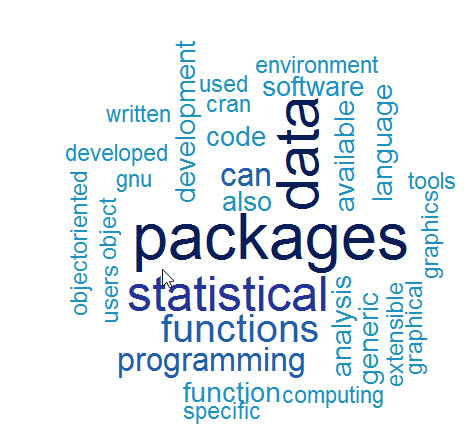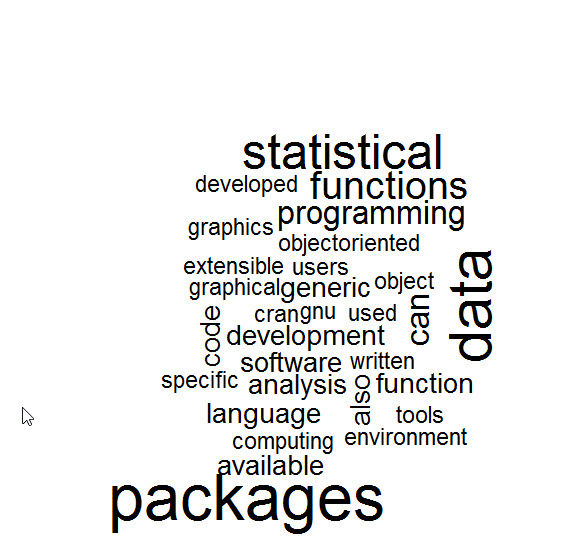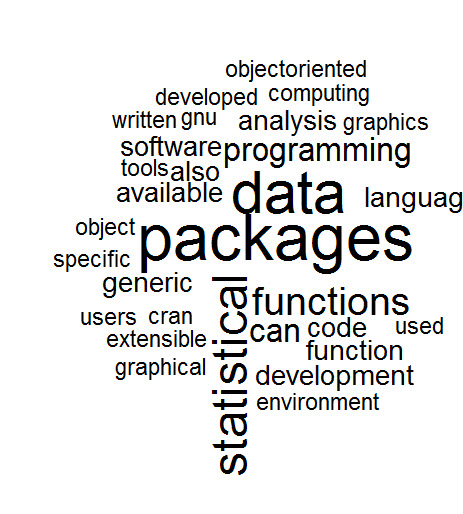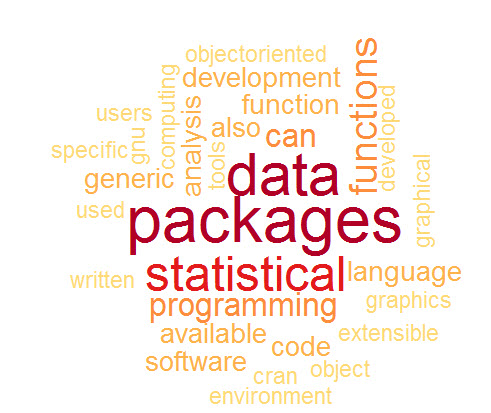# R: Creating a Word Cloud

Word Clouds are great visualization techniques for dealing with text analytics. The idea behind them is they display the most common words in a corpus of text. The more often a word is used, the larger and darker it is.Making a word cloud in R is relatively easy. The tm and wordcloud libraries from R’s CRAN repository is used to create one.

```library(tm)
library(wordcloud)

```

If you do not have either of these loaded on your machine, you will have to use the following commands

```install.packages("tm")
install.packages("wordcloud")```

Now in order to make a word cloud, you first need a collection of words. In our example I am going to use a text file I created from the Wikipedia page on R.

Now let’s load the data file.

```text <- readLines("rWiki.txt")
 "R is a programming language and software environment
 "The R language is widely used among statisticians and
 "Polls, surveys of data miners, and studies of scholarly
 "R is a GNU package. The source code for the R
 "General Public License, and pre-compiled binary versions
 "R is an implementation of the S programming language "
>```

Notice each line in the text file is an individual element in the vector –  text

Now we need to move the text into a tm element called a Corpus. First we need to convert the vector text into a VectorSource.

```wc <- VectorSource(text)
wc <- Corpus(wc)```

Now we need to pre-process the data. Let’s start by removing punctuation from the corpus.

`wc <- tm_map(wc, removePunctuation)`

Next we need to set all the letters to lower case. This is because R differentiates upper and lower case letters. So “Program” and “program” would treated as 2 different words. To change that, we set everything to lowercase.

`wc <- tm_map(wc, content_transformer(tolower))`

Next we will remove stopwords. Stopwords are commonly used words that provide no value to the evaluation of the text. Examples of stopwords are: the, a, an, and, if, or, not, with ….

`wc <- tm_map(wc, removeWords, stopwords("english"))`

Finally, let’s strip away the whitespace

`wc <- tm_map(wc, stripWhitespace)`

Now let us make our first word cloud

The syntax is as follows – wordcloud( words = corpus, scale = physical size, max.word = number of words in cloud)

`wordcloud(words = wc, scale=c(4,0.5), max.words=50)`Now we have a word cloud, let’s add some more elements to it.

random.order = False brings the most popular words to the center

`wordcloud(words = wc, scale=c(4,0.5), max.words=50,random.order=FALSE)`To add a little more rotation to your word cloud use rot.per

```wordcloud(words = wc, scale=c(4,0.5), max.words=50,random.order=FALSE,
rot.per=0.25)```

Finally, lets add some color. We are going to use brewer.pal.  The syntax is brewer.pal(number of colors, color mix)

```cp <- brewer.pal(7,"YlOrRd")
wordcloud(words = wc, scale=c(4,0.5), max.words=50,random.order=FALSE,
rot.per=0.25, colors=cp)```## One thought on “R: Creating a Word Cloud”

1.Shruthi Yadav

Can you provide articles on SVM, Time series and NaiveBayes algorithms in R